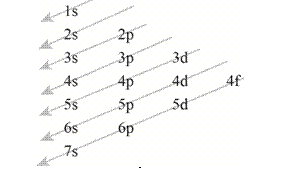Chapter 11, Problem 65QAP### Introductory Chemistry: A Foundati...

9th Edition
Steven S. Zumdahl + 1 other
ISBN: 9781337399425

#### Solutions

Chapter
Section### Introductory Chemistry: A Foundati...

9th Edition
Steven S. Zumdahl + 1 other
ISBN: 9781337399425
Textbook Problem
412 views

# For each of the following elements, indicate which set of orbitals is filled last. radium, Z = 88 c. gold, Z = 79 iodine, Z = 53 d. lead, Z = 82

Interpretation Introduction

(a)

Interpretation:

The set of orbitals that is filled last in the given element is to be stated.

Concept Introduction:

According to the Aufbau principle, “the electrons fill the atomic orbital of an atom that has lowest energy level, before occupying the higher levels of the atomic orbital of an atom.” The filling pattern of the electrons in the atomic orbitals of an atom is shown below.Figure 1.

Explanation

The electronic configuration of radium that has atomic number equal to Z=88 is shown as,

[Rn]7s2

As, the electronic configuration of radium is

(b)

Interpretation Introduction

Interpretation:

The set of orbitals that is filled last in the given element is to be stated.

Concept Introduction:

According to the Aufbau principle, ‘the electrons fill the atomic orbital of an atom that has lowest energy level, before occupying the higher levels of the atomic orbital of an atom.’ The filling pattern of the electrons in the atomic orbitals of an atom is shown below.Figure 1.

Interpretation Introduction

(c)

Interpretation:

The set of orbitals that is filled last in the given element is to be stated.

Concept Introduction:

According to the Aufbau principle, ‘the electrons fill the atomic orbital of an atom that has lowest energy level, before occupying the higher levels of the atomic orbital of an atom.’ The filling pattern of the electrons in the atomic orbitals of an atom is shown below.Figure 1.

Interpretation Introduction

(d)

Interpretation:

The set of orbitals that is filled last in the given element is to be stated.

Concept Introduction:

According to the Aufbau principle, ‘the electrons fill the atomic orbital of an atom that has lowest energy level, before occupying the higher levels of the atomic orbital of an atom.’ The filling pattern of the electrons in the atomic orbitals of an atom is shown below.Figure 1.

### Still sussing out bartleby?

Check out a sample textbook solution.

See a sample solution

#### The Solution to Your Study Problems

Bartleby provides explanations to thousands of textbook problems written by our experts, many with advanced degrees!

Get Started